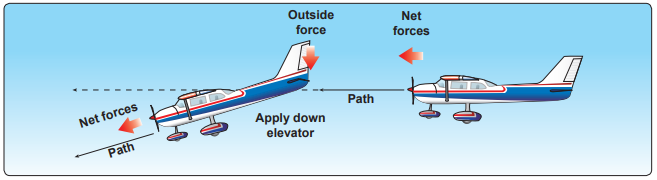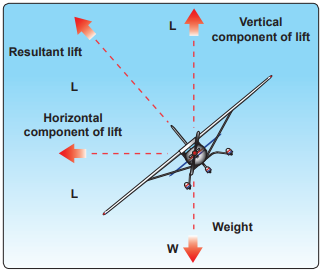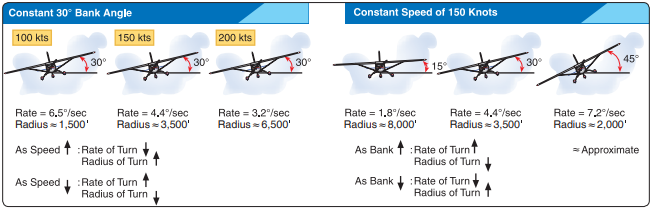top of page

# IFH Chapter 4

Updated: Apr 12, 2022

CHAPTER TITLE: Aerodynamic Factors

Below is a list of the figures (diagrams, charts, and pictures) from the IFH Chapter 4. They are listed in the order they are found in the Instrument Flying Handbook.

AUDIO RECORDING

FIGURE 4-1

The airfoil.FIGURE 4-2

Angle of attack and relative wind.FIGURE 4-3

The four forces and three axes of rotation.FIGURE 4-4

Newton’s First Law of Motion: the Law of Inertia.FIGURE 4-5

Newton’s Second Law of Motion: the Law of Momentum.FIGURE 4-6

Newton’s Third Law of Motion: the Law of Reaction.FIGURE 4-7

Koch chart sample.FIGURE 4-8

Relationship of lift to AOA.FIGURE 4-9

Thrust and power required curves.FIGURE 4-10

Regions of command.FIGURE 4-11

Region of speed stability.FIGURE 4-12

Plain and split flaps.FIGURE 4-13

Vortex generators.FIGURE 4-14

Forces in a turn.FIGURE 4-15

Turns.FIGURE 4-16FIGURE 4-17

Clear ice.FIGURE 4-18

Clear ice buildup with horns.FIGURE 4-19

Rime ice.FIGURE 4-20

Aerodynamic effects of icing.FIGURE 4-21

Effect of ice and frost on lift.FIGURE 4-22

Downward force on the tailplane.FIGURE 4-23

Ice on the tailplane.bottom of page